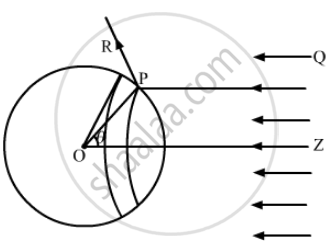Advertisement Remove all ads

# Consider the Situation Described in the Previous Problem. Show that the Force on the Sphere Due to the Light Falling on It is the Same Even If the Sphere is Not Perfectly Absorbing. - Physics

Sum

A sphere of radius 1.00 cm is placed in the path of a parallel beam of light of large aperture. The intensity of the light is 0.5 W cm−2. If the sphere completely absorbs the radiation falling on it, Show that the force on the sphere due to the light falling on it is the same even if the sphere is not perfectly absorbing.

Advertisement Remove all ads

#### Solution

Consider a sphere of centre O and radius OP. As shown in the figure, the radius OP of the sphere is making an angle θ with OZ. Let us rotate the radius about OZ to get another circle on the sphere. The part of the sphere between the circle is a ring of area 2pir^2sin θdθ.Consider a small part of area ΔA of the ring at point P.

Energy of the light falling on this part in time Δt ,

ΔU = I Δ t (ΔA cos θ)

As the light is reflected by the sphere along PR, the change in momentum ,

Δp = 2 (ΔU)/c cos θ = 2/c I Δ t (ΔA cos^2 θ)

Therefore , the force will be

(Δp)/(Δt) = 2/c I ΔA cos^2 θ

The Component of force on ΔA , along ZO , is

(Δp)/(Δt) cos θ = 2/c I ΔA cos^3 θ

Now , force action on the ring,

dF = 2/c I (2pir^2 sin  θ  dθ) cos^3 θ

The force on the entire sphere ,

F = ∫_0^(pi/2) (4pir^2I)/c cos^3 θ  sin  θ  dθ

= - ∫_0^(pi/2) (4pir^2I)/c cos^3 θd(cos θ)

= (pir^2I)/c

Is there an error in this question or solution?
Advertisement Remove all ads

#### APPEARS IN

HC Verma Class 11, Class 12 Concepts of Physics Vol. 2
Chapter 20 Photoelectric Effect and Wave-Particle Duality
Q 11 | Page 365
Advertisement Remove all ads
Advertisement Remove all ads
Share
Notifications

View all notifications

Forgot password?